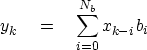Next: Negative time Up: SAMPLED DATA AND Z-TRANSFORMS Previous: Dissecting systems by factoring

## Convolution equation and program

What do we actually do in a computer when we multiply two Z-transforms together? The filter 2 + Z would be represented in a computer by the storage in memory of the coefficients (2, 1). Likewise, for 1 - Z, the numbers (1, -1) would be stored. The polynomial multiplication program should take these inputs and produce the sequence (2, -1, -1). Let us see how the computation proceeds in a general case, say(5) (6)
Identifying coefficients of successive powers of Z, we get(7)
In matrix form this looks like(8)
The following equation, called the convolution equation,'' carries the spirit of the group shown in (7):(9)
To be correct in detail when we associate equation (9) with the group (7), we should also assert that either the input xk vanishes before k=0 or Nb must be adjusted so that the sum does not extend before x0. These end conditions are expressed more conveniently by defining j=k-i in equation (9) and eliminating k getting(10)
A convolution program based on equation (10) including end effects on both ends, is convolve().
#       convolution:    Y(Z) = X(Z) * B(Z)
#
subroutine convolve( nb, bb, nx, xx, yy )
integer nb      # number of coefficients in filter
integer nx      # number of coefficients in input
# number of coefficients in output will be nx+nb-1
real    bb(nb)  # filter coefficients
real    xx(nx)  # input trace
real    yy(1)   # output trace
integer ib, ix, iy, ny
ny = nx + nb -1
call null( yy, ny)
do ib= 1, nb
do ix= 1, nx
yy( ix+ib-1) = yy( ix+ib-1) + xx(ix) * bb(ib)
return; end

Some details of the Ratfor programming language are given in an appendix, along with the subroutine zero(), which erases the space for the output.Next: Negative time Up: SAMPLED DATA AND Z-TRANSFORMS Previous: Dissecting systems by factoring
Stanford Exploration Project
10/21/1998